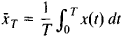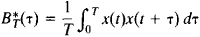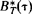# Statistical Analysis of Stochastic Processes

The following article is from The Great Soviet Encyclopedia (1979). It might be outdated or ideologically biased.

## Statistical Analysis of Stochastic Processes

the branch of mathematical statistics that deals with methods of handling and using statistical data concerning stochastic processes, that is, functions X(t) of time t that are determined by means of some experiment and that can take on different values in different experiments in a random manner. The value x(t) obtained for the stochastic process X(t) in the course of a single experiment is called a realization of the process. The statistical data on X(t) used in analyzing the process are generally the values of one or more realizations x(t) over a certain time interval or the values of some variables associated with X(t) —for example, the observed values of a process Y(t) that represents the sum of X(t) and some noise N(t) consisting of external noise and the errors in the measurement of the values of x(t).

With respect to applications, a very important class of problems in the statistical analysis of stochastic processes consists of problems of detecting a signal against a noise background. Such problems play an important role in radar. From the mathematical standpoint, these problems reduce to the testing of hypotheses. Here, the observed values of some function are used to determine which of the following two hypotheses holds: (1) the function is a realization of the sum of the noise N(t) and the signal X(t) of interest to the observer, or (2) the function is a realization of just the noise N(t). When the shape of the signal X(t) is not completely known, the problem of detection often involves the making of statistical estimates of the unknown signal parameters. For example, in radar problems it is very important to estimate the time of appearance of a signal; this time determines the distance to the object that produced the signal.

Problems of the statistical estimation of parameters also arise when, on the basis of observations of the values of the process X(t) over a certain time interval, it is required to estimate (1) the values of some parameters of the probability distribution of the random variables X(t), (2) the value of the process X(i) at a fixed moment in time t = r, (assuming that tx lies outside the time interval in which observations of the process were carried out), or (3) the value y(t1) of some auxiliary process Y(t) that is statistically associated with X(t) (seeSTOCHASTIC PROCESS, EXTRAPOLATION OF).

Finally, a number of problems in the statistical analysis of stochastic processes involve nonparametric statistical methods. In some cases, for example, it is required, on the basis of observations of the course of the process X(t), to estimate certain functions that characterize the probability distributions of the values of the process. Examples of such functions are the probability density of the variable X(t), the correlation function EX(t)X(s) of the process X(t), and, when X(t) is a stationary stochastic process, the spectral density/(X) of the process.

In solving problems of the statistical analysis of the stochastic process X(t), it is always necessary to make certain special assumptions about the statistical structure of the process—that is, to limit in some way the class of stochastic processes that will be considered. From the standpoint of the statistical analysis of stochastic processes, it is very useful to assume that the given process X(i) is a stationary process. If the value of a single realization x(t) within the time interval 0< t < T is known, the assumption of stationarity permits a number of statistical conclusions to be made regarding the probability characteristics of the process X(t). In particular, the mean valueof a stationary stochastic process X(t) is, under extremely broad conditions, a consistent estimate of the mathematical expectation BX(t) = w; in other words, as T →∞ xT converges to the true value of the estimated quantity m. Similarly, the sample correlation functionwhere τ > 0, is under extremely broad conditions, a consistent estimate of the correlation function

B(τ) = EX(t)X(t + τ)

It should be noted, however, that the periodogram IT(λ) of the process X(t) [the periodogram is the Fourier transform of] is not a consistent estimate of the spectral density f(λ), which is the Fourier transform of B(λ). For large values of T, IT(λ) behaves extremely irregularly; as T → ∞, IT(λ) does not tend to any limit. The statistical analysis of stochastic processes therefore involves a number of special methods for making consistent estimates of the spectral density f(λ) on the basis of the observed values of a single realization of the stationary process X(f); most of the estimates are based on the smoothing of the periodogram of the process over a comparatively narrow range of frequencies λ.

In studying the statistical properties of estimates of the probabilistic quantities characterizing stationary stochastic processes, it is very useful to make additional assumptions regarding the nature of X(t). For example, it may be assumed that all finite-dimensional distributions of the values of X(t) are normal probability distributions. Much progress has also been made in research on the statistical analysis of stochastic processes where it is assumed that the process X(t) under study is a particular type of Markov process, a component of a multidimensional Markov process, or a component of a multidimensional process that satisfies a certain system of stochastic differential equations.

### REFERENCES

Jenkins, G., and D. Watts. Spektral’nyi analiz i ego prilozheniia, fascs. 1–2. Moscow, 1971–72. (Translated from English.)
Hannan, E. Analiz vremennykh riadov. Moscow, 1964. (Translated from English.)
Hannan, E. Mnogomernye vremennye riady. Moscow, 1974. (Translated from English.)
Liptser, R. Sh., and A. N. Shiriaev. Statistika sluchainykh protsessov (netineinaiafil’tratsiia ismezhnye voprosy). Moscow, 1974.

A. M. IAOLOM